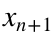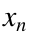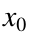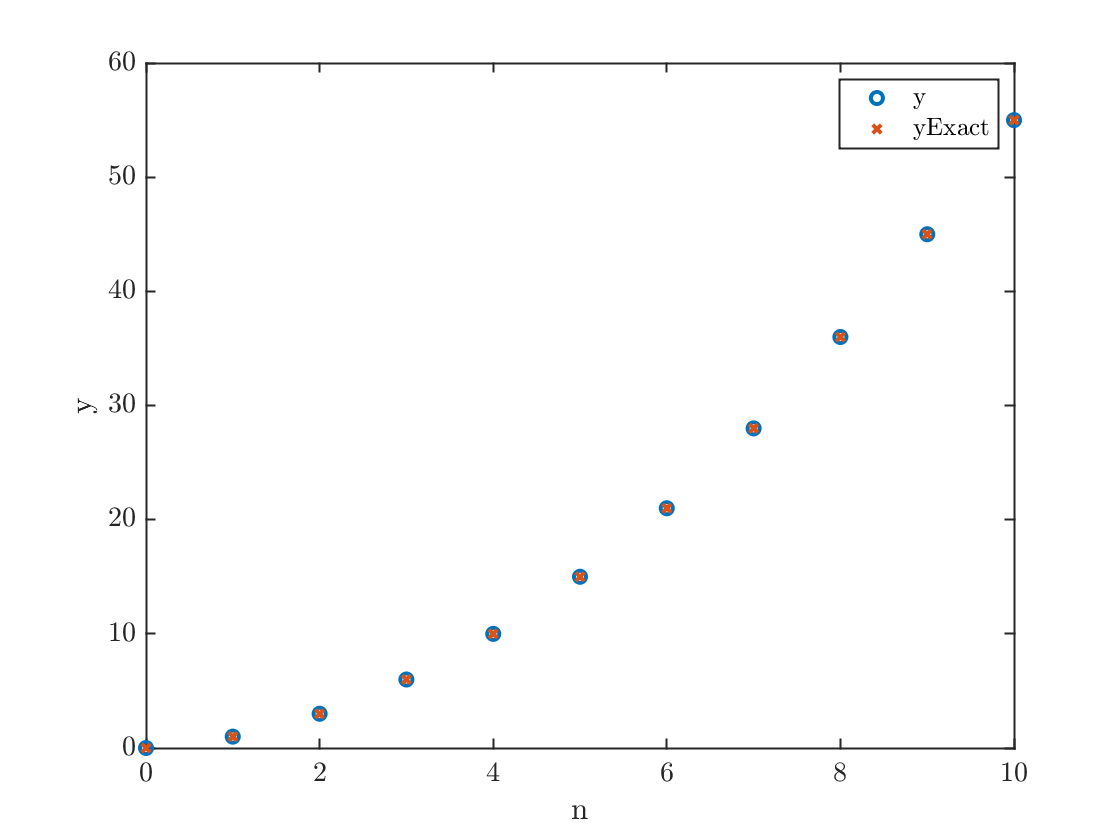# Math 222 MATLAB Supplementary lecture

## Part 1: For loops and recurrence relations

Any formula which generates a sequence and givesin terms ofis called a recurrence relation. Of course such a formula needs an initial value. A very simple recurrence relation isThis define a sequence of valuesN = 10;
n=0:N; % note that this starts from n=0
y = zeros(1,N+1);
y(1) = 0;
for j=1:N
y(j+1) = y(j) + j;
end
We know, from first semester calculus (NJIT Math 111), that the exact solution to this recurrence isyExact=n.*(n+1)/2; % Note the use of .* to multiply arrays!
We plot both of these on the same axis. Note the plotting syntax, including both the plots of both y and yExact, is not taught in the Onramp, but how it works should be obvious
plot(n,y,'o',n,yExact,'x')
xlabel('n');ylabel('y')
legend('y','yExact')